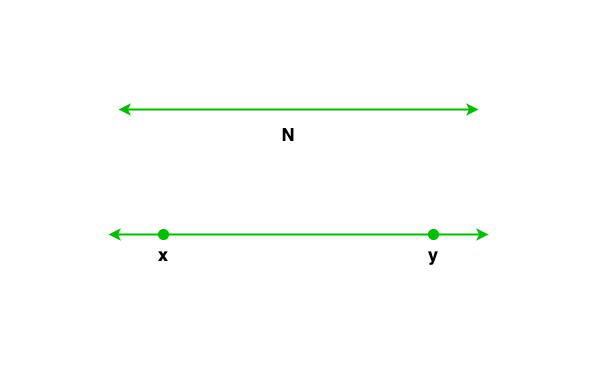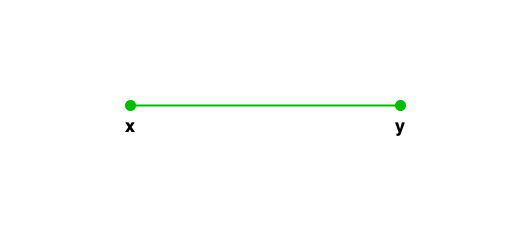# Difference between a Line and Line segment

• Last Updated : 16 Dec, 2021

Geometry is the study of shapes or geometrical figures. All the shapes we observe in geometry are made up by the joining of a number of lines. And, lines are the collection of points. This interrelation of points, lines, and shapes makes the study of geometrical figures equally interesting. The article also observes the same topics of geometrical figures with details about points, lines, and line segments along with their properties. The article is intended to show the difference between a line and a line segment.

Points

Points are building blocks of geometrical figures. All the geometrical structures drawn till now are the combination of uncountable numbers of points. Points are structures with no dimensions. They do not possess length, breadth, or height. The points are symbolically denoted by a dot(.). And, the joining of these dots helps to draw a shape.

Lines

Lines are the geometrical figure with non-terminating ends. A line runs in two opposite directions endlessly. Both points of a line can be extended to infinity. Lines are roughly denoted or symbolized by any two points lying on the line.Properties of lines:

• A line extends up to infinity in both directions, which means it has infinite length.
• They do not consist of endpoints.
• A line is a one-dimensional geometrical figure, that is it has only length but no thickness or breadth.
• At any point, two lines intersecting each other will cross at a single common point.

Line segments

The line is non-ending but it can be fractionally divided into different segments. These segments are known as the line segment which has two endpoints. It is a part of the line that connects two points lying on it. The portion of a line segment is bounded in between two endpoints, in which all the points lying in between these endpoints become a part of the line segment.Properties of a line segment:

• A line segment is not a complete line but a fractional part of a line with two distinct endpoints.
• A line segment has two fixed endpoints.
• The length of a line segment is measurable as it has fixed ends.
• A line segment is symbolically represented by ‘_’ or### Sample Questions

Question 1. Name the five types of lines we observe in geometry.

Solution:

The five types of lines are

• Straight line
• Curved line
• Parallel line
• Perpendicular line
• Intersecting line

Question 2. What are rays?

Solution:

A ray is a geometrical figure or part of a line that has a fixed starting point but does not have an end point.

Question 3. How many dimensions does the line have?

Solution:

A line is a uni-dimensional geometrical figure which only has length.

Question 4. Which type of line changes its direction?

Solution:

Curved lines have the property to change directions.

My Personal Notes arrow_drop_up